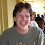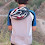## Friday, July 19, 2013

### A(nother) nice use of Gen Functions

In a prior post I tried to give a simple example of a proof that uses Gen Functions where there was no other way to do it. For better or worse, before I posted it, my HS student Sam found a better way and I posted both proofs.

I have another example. Noga Alon showed this to be over dinner at the Erdos 100th Bday conference. (He claims that the proof he showed me is NOT his but he doesn't know whose it is. I will still call it Noga's Proof for shorthand.)

Let

A+A = { x+y : x,y ∈ A}

A+*A = { x+y : x,y ∈ A and x ≠ y }

We take both to be multisets.

Assume A is a set of natural numbers. When does A+*A determine A?

If A is of size 2 then NO, A+*A does not determine A as we could have x+y=5 but not know if A is {1,4} or {2,3}.

What if A is of size 3? Then YES:

First determine S=((x+y)+(x+z)+(y+z))/2=x+y+z.

Then determine

x = S - (y+z)

y = S - (x+z)

z = S - (x+y)

What if A has four elements? Does there exists A,B of size 4, different, such that A+*A=B+*B?

YES:

A = {1,4,12,13}

B = {2,3,11,14}

For which n does does A+*A, where A is of size n, determine A?

Selfridge and Strauss showed that this happens iff n is NOT a power of two. I have a write up Noga's proof. The original proof, in this paper, does not use gen functions and also applies to sets of complex numbers. I think Noga's proof can be modified to apply here. Which proof is better? A matter of taste; however, Noga's proof can be sketched on a greasy paper placemat in an outdoor restaurant in Budapest while the original proof cannot.

1.That's a nice proof! As for complex numbers, just having the proof for natural numbers seems to give away everything.

Claim 1: The result holds for integers.
Proof: Translate A so that it now holds natural numbers, and notice that no information is lost.

Claim 2: The result holds for Z^k.
Proof idea: Let A be a set of k-vectors, with maximum element M (in absolute value). Treat the vectors in A as (something like) base-(4M+1) representations of integers, and let A' be the corresponding set of integers. Given A +* A, we can translate to A' +* A', then recover A', and then recover A.

Claim 3: The result holds for complex numbers.
Proof: View C as a vector space over Z and apply claim 2. (Note that this doesn't use the axiom of choice, since a finite set A only generates a finite-dimensional vector space over Z.)

2.So, one thing I didn't understand in your proof (or Noga's proof or whatever): In page 3, when you divide both sides of Equation by (x-1)^m, you can only do that if $x$ is not 1. So, you get a condition on the side (that you don't mention) and that says the resulting equation is true for $x \neq 1$. Now, you put $x$ to be 1 which is in direct contradiction with that condition on the side and you get to your desired result this way.

While the final result happens to be the same as the original way of doing it, your proof (or Noga's proof or ...) seems not to be valid.

Please tell me if I'm missing something ....